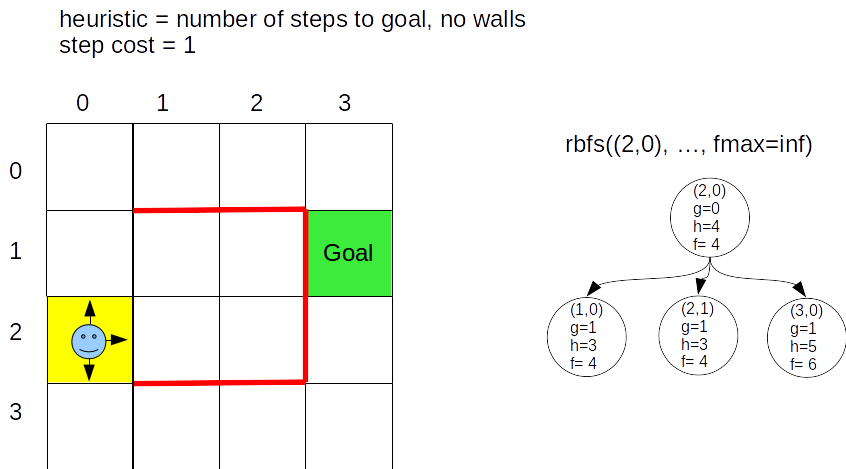An admissible heuristic is one that never overestimates the cost of the minimum cost path from a node to the goal node. So, a heuristic is specific to a particular state space, and also to a particular goal state in that state space. It must be admissible for all states in that search space.

To help remember whether it is "never overestimates" or "never underestimates", just remember that an admissible heuristic is too optimistic. It will lead A* to search paths that turn out to be more costly that the optimal path. It will not prevent A* from expanding a node that is on the optimal path by producing a heuristic $h$ value that is too high.

A stronger requirement on a heuristic is that it is consistent, sometimes called monotonic. A heuristic $h$ is consistent if its value is nondecreasing along a path. Mathematically, a heuristic $h$ is consistent if for every node $n$ of a parent node $p$,

$$h(p) \le h(n) + \mathrm{stepcost}(p,n)$$

Every consistent heuristic must be admissible. (How would you show this?) Sometimes showing a heuristic is consistent is a good way of proving it is admissible.

# Example Robot Search Problem¶# Proof of A* Optimality¶

Section 3.5.2 describes one proof of A* optimality that relies on $h$ being consistent. It proceeds as follows.

For a goal node $n$, $f(n)$ is the true cost of the path from the start node to this goal node; $f(n) = g(n) + h(n) = g(n) + 0$ because $h(n)=0$ for goal nodes.

If A* expands nodes in nondecreasing order of $f(n)$, then the first goal node selected for expansion must be an optimal solution, because for goal nodes $f(n)$ is the true cost of path to this goal node.

We must show that A* expands nodes in nondecreasing order.

Suppose node $n$ is a child of node $p$. Then

\begin{align*} f(n) &= g(n) + h(n)\\ &= g(p) + \mathrm{stepcost}(p,n) + h(n) & \text{ because } g(n) = g(p) + \mathrm{stepcost}(p,n)\\ &\ge g(p) + h(p) & \text{ assuming } h \text{ is consistent, meaning }\\ & & ~~~~~~~~~ h(p) \le h(n) + \mathrm{stepcost}(p,n) \\ &= f(p) \end{align*}

so

\begin{align*} f(n) &\ge f(p) \end{align*}

In other words, if $h(n)$ is consistent for all $n$, then the values of $f(n)$ along any path are nondecreasing.

Now we must show that whenever A* selects a node $n$ for expansion, an optimal path to that node has been found. If this were not true, another node $m$ must exist on the unexpanded (frontier) list through which a better path exists from start node to node $n$. But, this node would have a lower $f$ value and would be selected next instead of node $n$, a contradiction.

Here is another way of proving that the optimal path to a goal is found. It only assumes the heuristic is admissible.

Consider this situation.$a$ and $b$ both satisfy the goal function, but $b$ is optimal, $a$ is not.

Assume $a$ is selected before $n$. This will lead to a contradiction, showing this assumption is wrong.

Let $f^*$ be the cost of the optimal path to $b$. Let $n$ be a parent node to $b$ on the optimal path to $b$.

If $h$ is admissible,

\begin{align*} h(n) & \le \text{true optimal cost from }n\text{ to a goal.}\\ g(n) + h(n) &\le g(n) + \text{true optimal cost from }n\text{ to a goal.}\\ f(n) &\le f^* \end{align*}

Since we are assuming $a$ is selected before $n$,

\begin{align*} f(a) & \le f^*\\ g(a) + h(a) & \le f*\\ g(a) &\le f^* &\text{because }a\text{ is a goal node so }h(a)=0 \end{align*}

This means we have just proved that the true cost to $a$, $g(a)$, is less than the optimal cost to a goal node, a contradiction. So, our assumption that a suboptimal goal is chosen was wrong.

# Optimally Efficient¶

No other algorithm that extends search paths from the start node and uses the same heuristic information will expand fewer nodes that A*.

However, maintaining the list of unexpanded frontier nodes can quickly consume all storage. This is why we focus on the recursive-best-first-search version of A*. Its iterative-deepening strategy of throwing away and regenerating nodes reduces the maximum number of nodes stored at any point of the algorithm. Its space complexity is linear in the depth of the deepest optimal solution. Its time complexity is hard to characterize as it depends on the accuracy of the heuristic function.

This form of A* throws away too many nodes to be as efficient in time as it can be. Alternatives include the simplified memory-bounded A*, SMA*, algorithm. SMA* proceeds like a graph-based search maintaining the unexplored frontier list. When it runs out of memory, it deletes the node with the worst $f$ value and backs that value up to the deleted node's parent.

# Heuristic Functions¶

Okay, so we must have admissible heuristic functions, ones that never overestimate the true remaining cost to a goal. Are some admissible heuristics better than others?

We want ones that mislead A* least often. A heuristic will mislead if its estimate of remaining cost to goal is much, much lower than the true cost. So, we want heuristics that are as close as possible to the true remaining cost for all nodes without going over the true cost, and thus overestimate and are not admissible. A heuristic $h_1$ is better than $h_2$ if $h_1(n) \ge h_2(n)$ for all $n$. $h_1$ is said to "dominate" $h_2$.

# A* Practice Exercise¶

Complete the following A* search tree before next class. Answer will be discussed in class.